# Canonical Polyhedra

The canonical forms of polyhedra with 4 to 9 faces

Originator: Ed Pegg Jr (Wolfram Research)

## Examples

### Basic Examples

Retrieve the ResourceObject:

 In:=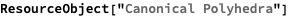Out=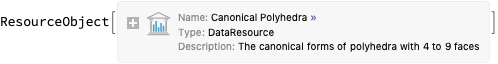View the data:

 In:=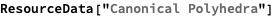Out=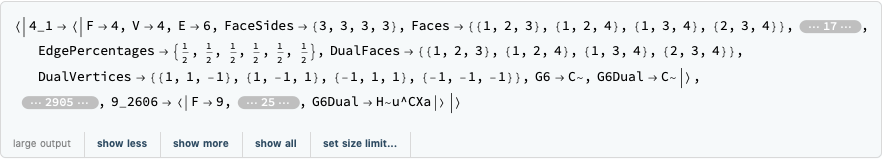View the data for the canonical form of the regular octahedron:

 In:=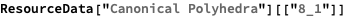Out=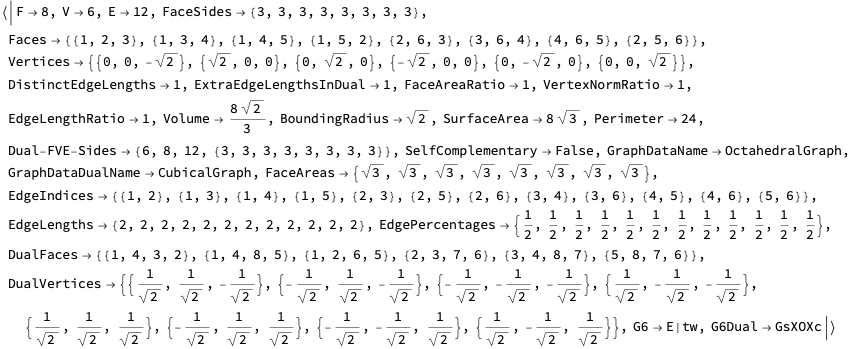View a nonahedron “9_7” with a unit sphere, showing the tangency to the edges:

 In:=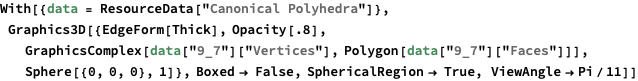Out=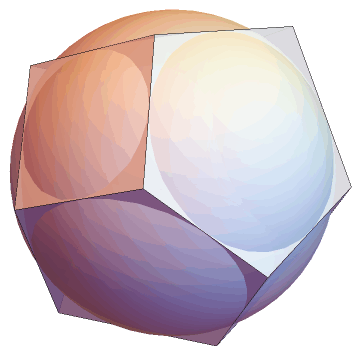See the seven canonical hexahedra and their duals:

 In:=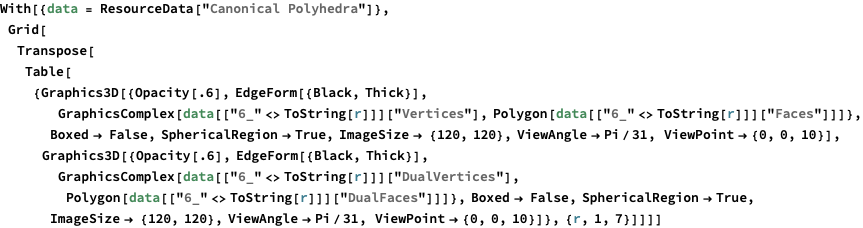Out=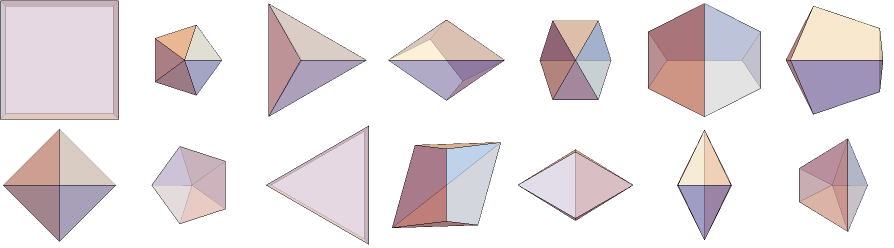Render the 34 canonical heptahedra, viewed from above:

 In:=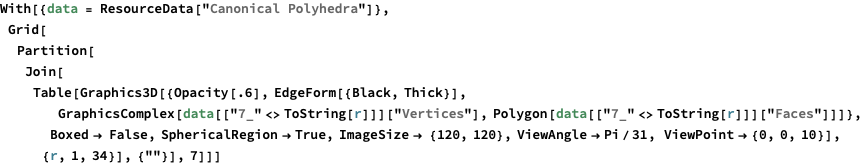Out=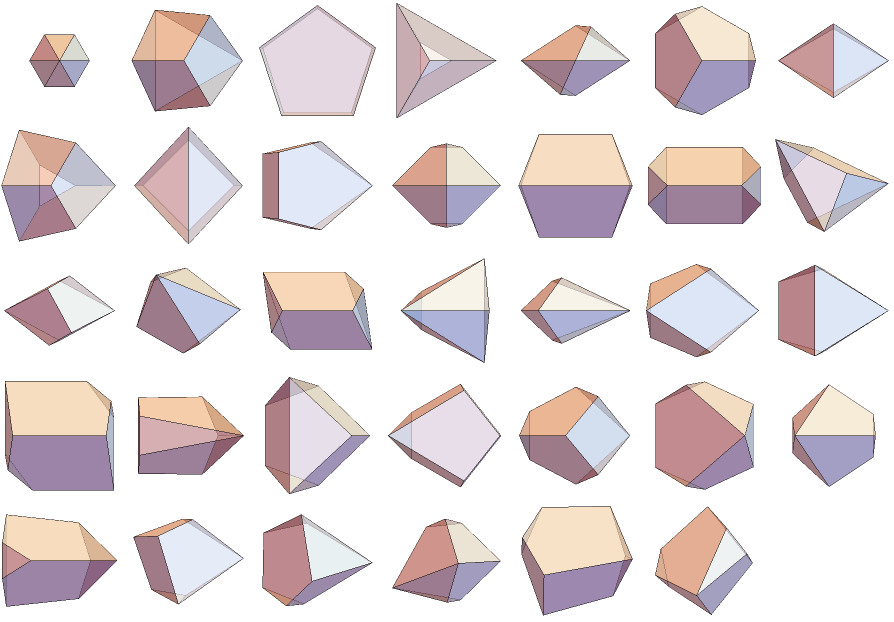Draw the Schlegel diagrams of the 34 heptahedra:

 In:=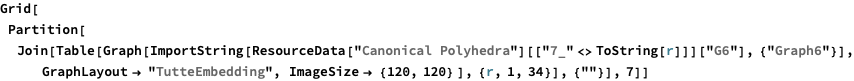Out=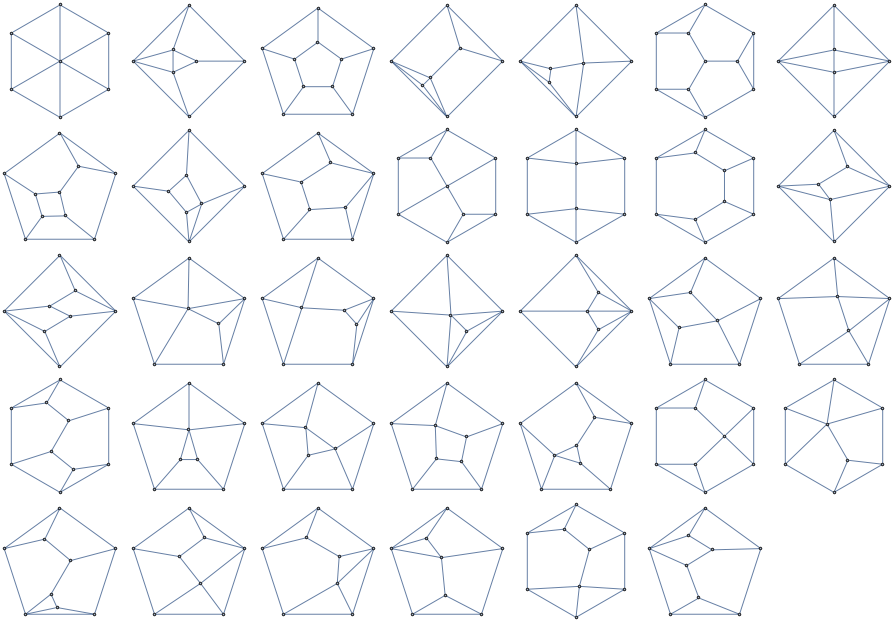The pyramid built from an n-gon is self-complementary or self-dual. Here are 35 of the 70 other self-dual polyhedra within the dataset, each shown with the dual. Notice how the edges are always perpendicular to each other:

 In:=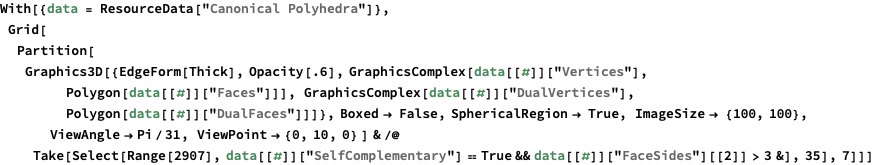Out=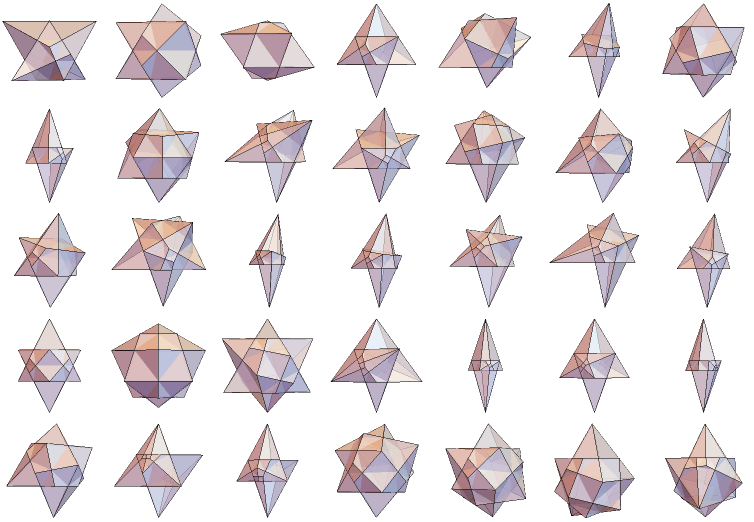Wolfram Research, "Canonical Polyhedra" from the Wolfram Data Repository (2017) https://doi.org/10.24097/wolfram.98862.data